# Confidence IntervalsAn interval of 4 plus or minus 2

A Confidence Interval is a range of values we are fairly sure our true value lies in.### Example: Average Height

We measure the heights of 40 randomly chosen men, and get a mean height of 175cm,

We also know the standard deviation of men's heights is 20cm.

The 95% Confidence Interval (we show how to calculate it later) is:

175cm ± 6.2cmThis says the true mean of ALL men (if we could measure all their heights) is likely to be between 168.8cm and 181.2cm.

But it might not be!

The "95%" says that 95% of experiments like we just did will include the true mean, but 5% won't.

So there is a 1-in-20 chance (5%) that our Confidence Interval does NOT include the true mean.

## Calculating the Confidence Interval

Note: we should use the standard deviation of the entire population, but in many cases we won't know it.

We can use the standard deviation for the sample if we have enough observations (at least n=30, hopefully more).

Using our example:

• number of observations n = 40
• mean X = 175
• standard deviation s = 20

Step 2: decide what Confidence Interval we want: 95% or 99% are common choices. Then find the "Z" value for that Confidence Interval here:

 Confidence Interval Z 80% 1.282 85% 1.440 90% 1.645 95% 1.960 99% 2.576 99.5% 2.807 99.9% 3.291

For 95% the Z value is 1.960

Step 3: use that Z value in this formula for the Confidence Interval

X  ±  Zs√n

Where:

• X is the mean
• Z is the chosen Z-value from the table above
• s is the standard deviation
• n is the number of observations

And we have:

175 ± 1.960 × 20√40

Which is:

175cm ± 6.20cm

In other words: from 168.8cm to 181.2cm

The value after the ± is called the margin of error

The margin of error in our example is 6.20cm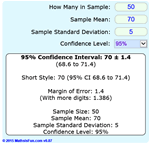## Calculator

We have a Confidence Interval Calculator to make life easier for you.

## Simulator

We also have a very interesting Normal Distribution Simulator. where we can start with some theoretical "true" mean and standard deviaition, and then take random samples.

It helps us to understand how random samples can sometimes be very good or bad at representing the underlying true values.

## Another Example### Example: Apple Orchard

Are the apples big enough?

There are hundreds of apples on the trees, so you randomly choose just 46 apples and get:

• a Mean of 86
• a Standard Deviation of 6.2

So let's calculate:

X  ±  Zs√n

We know:

• X is the mean = 86
• Z is the Z-value = 1.960 (from the table above for 95%)
• s is the standard deviation = 6.2
• n is the number of observations = 46

86 ± 1.960 × 6.2√46 = 86 ± 1.79

So the true mean (of all the hundreds of apples) is likely to be between 84.21 and 87.79

### True Mean

Now imagine we get to pick ALL the apples straight away, and get them ALL measured by the packing machine (this is a luxury not normally found in statistics!)

And the true mean turns out to be 84.9

Let's lay all the apples on the ground from smallest to largest: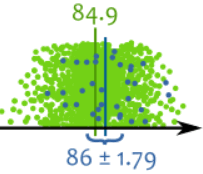Each apple is a green dot,
except our observations which are blue

Our result was not exact ... it is random after all ... but the true mean is inside our confidence interval of 86 ± 1.79 (in other words 84.21 to 87.79)

Now the true mean might not be inside the confidence interval, but in 95% of the cases it will be!

95% of all "95% Confidence Intervals" will include the true mean.

Maybe we had this sample, with a mean of 83.5: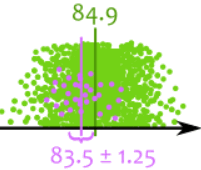Each apple is a green dot,
our observations are marked purple

That does not include the true mean. Expect that to happen 5% of the time for a 95% confidence interval.

So how do we know if the sample we took is one of the "lucky" 95% or the unlucky 5%? Unless we get to measure the whole population like above we simply don't know.

This is the risk in sampling, we might have a bad sample.

## Example in Research

Here is Confidence Interval used in actual research on extra exercise for older people: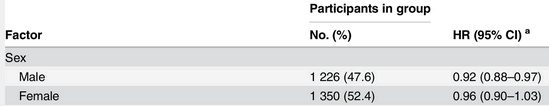What is it saying? Looking at the "Male" line we see:

• 1,226 Men (47.6% of all people)
• had a "HR" (see below) with a mean of 0.92,
• and a 95% Confidence Interval (95% CI) of 0.88 to 0.97 (which is also 0.92±0.05)

"HR" is a measure of health benefit (lower is better), so that line says that the true benefit of exercise (for the wider population of men) has a 95% chance of being between 0.88 and 0.97

* Note for the curious: "HR" is used a lot in health research and means "Hazard Ratio" where lower is better, so an HR of 0.92 means the subjects were better off, and 1.03 means slightly worse off.

## Standard Normal Distribution

It is all based on the idea of the Standard Normal Distribution, where the Z value is the "Z-score"

For example the Z for 95% is 1.960, and here we see the range from -1.96 to +1.96 includes 95% of all values: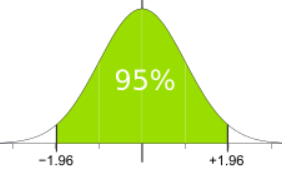From -1.96 to +1.96 standard deviations is 95%

Applying that to our sample looks like this: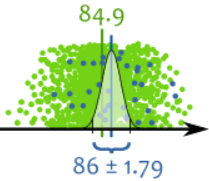Also from -1.96 to +1.96 standard deviations, so includes 95%

## Conclusion

The Confidence Interval is based on Mean and Standard Deviation. Its formula is:

X  ±  Zs√n

Where:

• X is the mean
• Z is the Z-value from the table below
• s is the standard deviation
• n is the number of observations
 Confidence Interval Z 80% 1.282 85% 1.440 90% 1.645 95% 1.960 99% 2.576 99.5% 2.807 99.9% 3.291